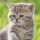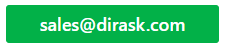Home
IT Knowledge
IT Career
Inspiration
Languages
EN

# JavaScript - calculate cos in degrees

3 points
Created by:Kate_C
19800

I prepared super simple example to calculate cos in degrees in JavaScript and decided to share with others. So in order to use this example we just need to change value of deg variable to some other value (in degrees).

``````// ONLINE-RUNNER:browser;

function calculateCos(deg) {
var rad = (Math.PI / 180) * deg;

}

var deg = 30;
var y = calculateCos(deg);

// cos(30 deg) = 0.8660254037844387
console.log('cos(' + deg + ' deg) = ' + y);``````

We can change deg to other valus. Examples of common calculations:

``````cos(0 deg) = 1
cos(30 deg) = 0.8660254037844387
cos(45 deg) = 0.7071067811865476
cos(60 deg) = 0.5000000000000001
cos(90 deg) = 6.123233995736766e-17``````

I prepated similar post but for calculation sin in degrees: